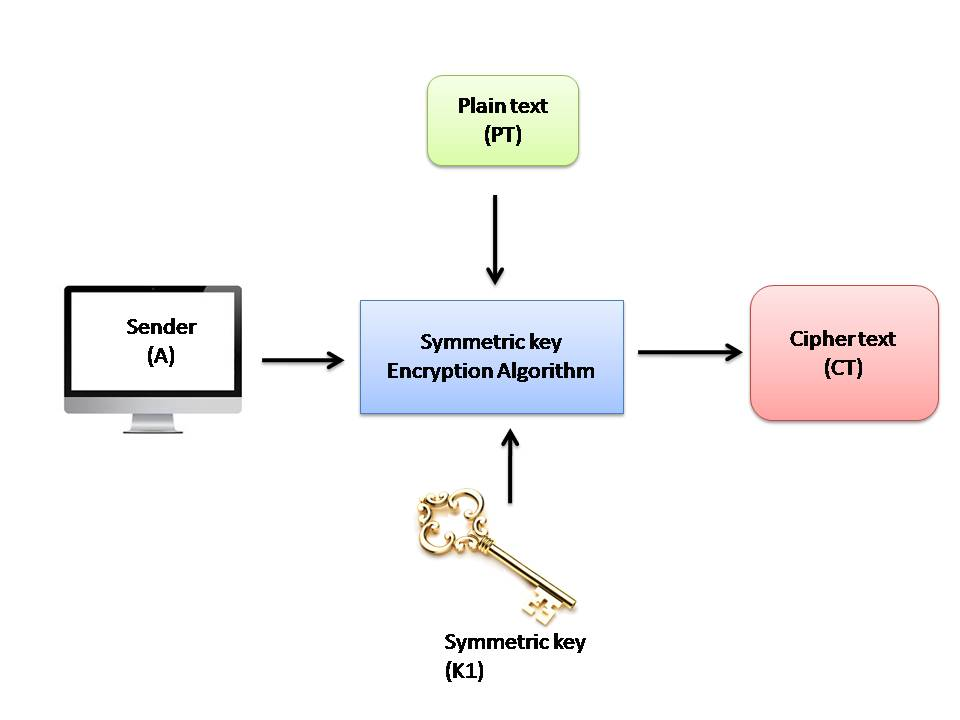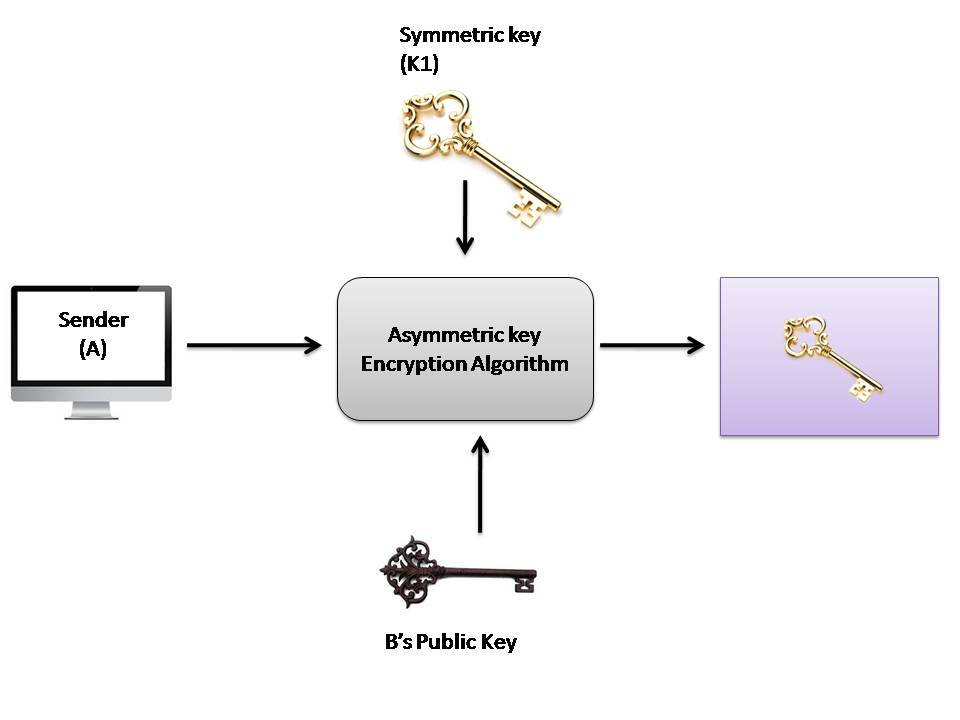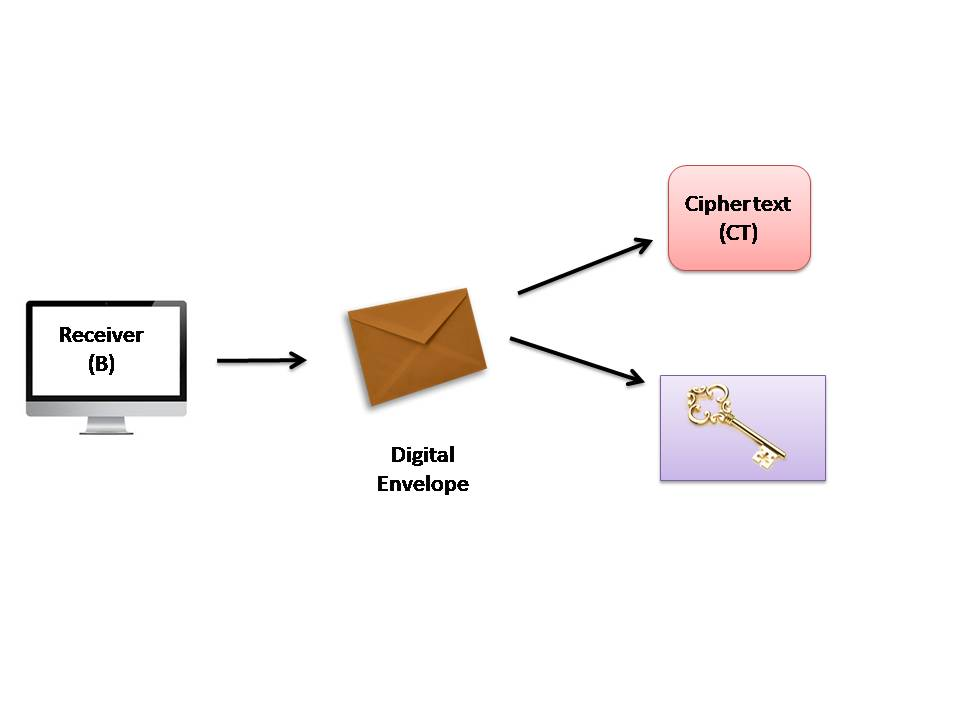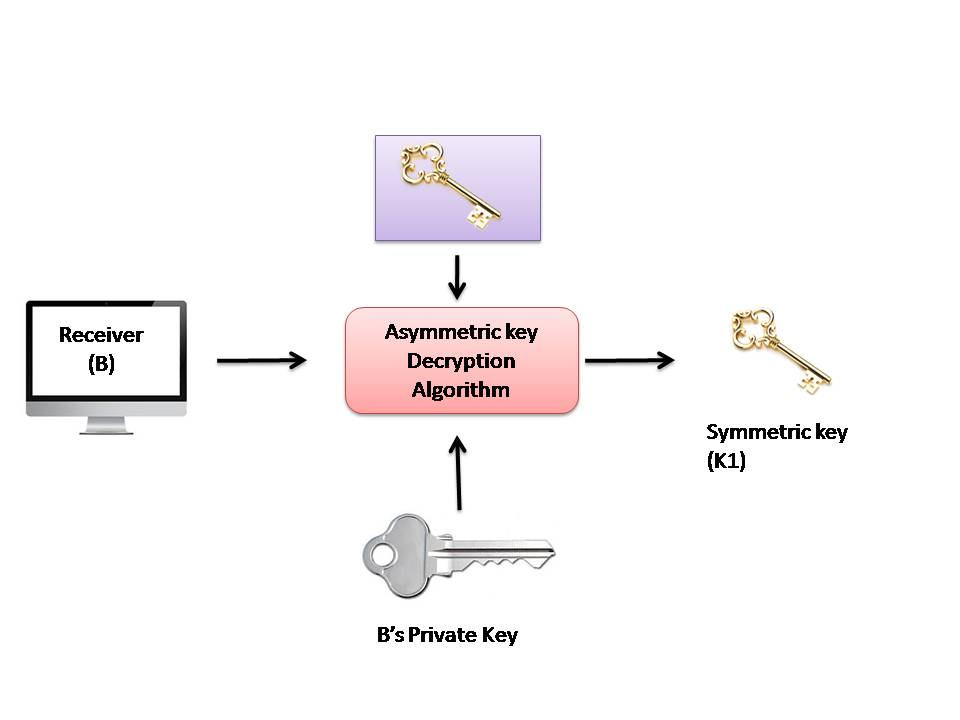• Indeed, in practice, symmetric-key cryptography and asymmetric-key cryptography are combined to have a very efficient security solution. The way it works is as follows, assuming that A is the sender of message and B is its receiver.
1. A’s computer encrypts the original plain-text message (PT) with the help of a standard symmetric key cryptography algorithm, such DES, IDEA or RC5, etc. this produces a cipher-text message (CT) as shown in Fig. below. The key used in this operation (K1) is called one-time symmetric key, as it is used once and then discarded.2. We would now think, we are back to square one! We have encrypted the plain text (PT) with a symmetric-key operation. We must now transport this one-time symmetric key (K1) to the server so that the server can decrypt the cipher text (CT) to get back the original plain-text message (PT). Does this not again lead us to the key-exchange problem? Well, a novel concept is used now. A now takes the one-time symmetric key of step 1 (i.e. K1), and encrypts K1 with B’s public key (K2). This process is called key wrapping of the symmetric key, and is shown in fig. below. We have shown that the symmetric key K1 goes inside a logical box, which is sealed by B’s public key (i.e. K2).3. . Now, A puts the cipher text CT1 and the encrypted symmetric key together inside a digital envelope. This is shown in fig. below4. The sender (A) now sends the digital envelope [which contains the cipher text (CT) and the onetime symmetric key (K1) encrypted with B’s public key, (K2)] to B using the underlying transport mechanism (network). This is shown in fig .we do not show the contents of the envelope, and assume that the envelope contains the two entities, as discussed.5. . B receives digital envelope and opens it . After B opens this digital envelope, he gets 2 things  first is cipher text (CT) and another one is the one-time session key (K1) which is encrypted using B’s public  key (K2). This is shown in fig. below.6. . B now uses the same asymmetric-key algorithm as was used by A and her private key (K3) to decrypt (i.e. open up) the logical box that contains the symmetric key (K1), which was encrypted with B’s public key (K2). This is shown in fig. below. This the output of the process is the one-time symmetric key K1.7.  Finally, B applies the same symmetric-key algorithm as was used by A, and the symmetric key K1 to decrypt the cipher text (C1). This process yields the original plain text (PT), as shown in fig. below.